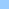# vectorShape_draw_line

how to draw a line using a vectorShape member
draw line vectorshapevectorShape draw line ```Hope this isn't too late. Here's a vector based solution to the line drawing question. Simply create a vertival vector line with 2 points; doesn't matter how tall you make it. Set the registration point of the member to be the bottom control point. Place it on the stage and attach this behavior to it. The rotation code came from Kevin Dowd, I just added a little thouch of Pythagoras math. property spritenum, pMyH, pMyV on beginSprite me pMyH = sprite(spritenum).locH pMyV = sprite(spritenum).locV end on exitframe me tempPos = the mouseloc x = (- pMyH + tempPos) y = (- pMyV + tempPos) * -1 rads = atan(x,y) deg = radToDegs (me, rads) newH = tempPos newV = tempPos deltaH = abs(newH - pMyH) deltaV = abs(newV - pMyV) -- Pythagoras currDistance = sqrt((deltaH * deltaH) + (deltaV * deltaV)) sprite(spritenum).height = currDistance sprite(spritenum).rotation = deg end on radToDegs me, theSum return theSum / pi() * 180 end Let me know if you need me to send you a sample movie. Pat Knoff ```Home shock + cgi Bits 'n pieces Director Lingo ShockLets Contact